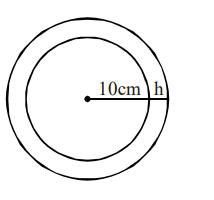# A spherical iron ball of radius 10 cm is coated with a layer ofQuestion:

A spherical iron ball of radius $10 \mathrm{~cm}$ is coated with a layer of ice of uniform thickness that melts at a rate of $50 \mathrm{~cm}^{3} / \mathrm{min}$. When the thickness of the ice is $5 \mathrm{~cm}$, then the rate at which the thickness (in $\mathrm{cm} / \mathrm{min}$ ) of the ice decreases, is :

1. $\frac{1}{9 \pi}$

2. $\frac{5}{6 \pi}$

3. $\frac{1}{18 \pi}$

4. $\frac{1}{36 \pi}$

Correct Option: , 3

Solution:$\mathrm{V}=\frac{4}{3} \pi\left((10+\mathrm{h})^{3}-10^{3}\right)$

$\frac{\mathrm{dV}}{\mathrm{dt}}=4 \pi(10+\mathrm{h})^{2} \frac{\mathrm{dh}}{\mathrm{dt}}$

$-50=4 \pi(10+5)^{2} \frac{\mathrm{dh}}{\mathrm{dt}}$

$\Rightarrow \frac{\mathrm{dh}}{\mathrm{dt}}=-\frac{1}{18} \frac{\mathrm{cm}}{\mathrm{min}}$